# Concatenate Date in Excel & Google Sheets

This tutorial will demonstrate how to concatenate a date with another string of text in Excel and Google Sheets.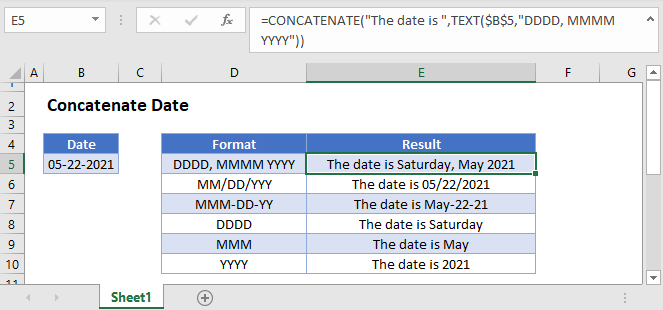## Join Date with Text

If you attempt to concatenate (join) a date with text, the date will be displayed as a number:

``=CONCATENATE(B3,C3)``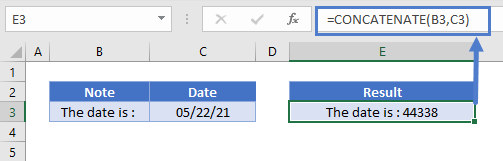This is because Excel stores dates as serial numbers; when you join a date with text, the cell formatting is not carried over.

### TEXT Function

The TEXT Function allows you to display a number as text with specified formatting. You can use the TEXT Function to set date formatting. Here’s an example with m/d/yyyy formatting:

``=TEXT(B3,"M/D/YYYY")``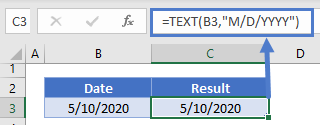Here is an example with MMMM D, YYYY formatting:

``=TEXT(B3,"mmmm d, yyyy")``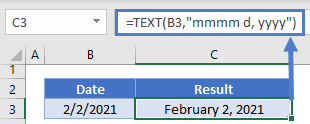### Create Date Range

You can join together two dates to create a date range using two text functions:

``=TEXT(B3, "m/d/yyyy")&" to " &TEXT(C3, "m/d/yyyy")``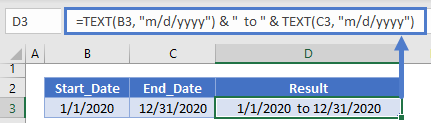Notice that this maintains the date formatting.

By adding in IF Statements you can create a smarter formula that won’t display blank dates:

``=IF(B3<>"",TEXT(B3, "m/d/yyyy")&" to " &TEXT(C3, "m/d/yyyy"),TEXT(C3,"m/d/yyyy") )``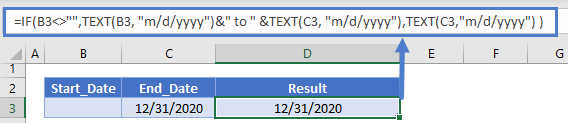or

``=TEXT(B3, "m/d/yyyy") & IF(C3<>"", " to " & TEXT(C3, "m/d/yyyy"), "")``

## Concatenate Date in Google Sheets

All of the above examples work exactly the same in Google Sheets as in Excel.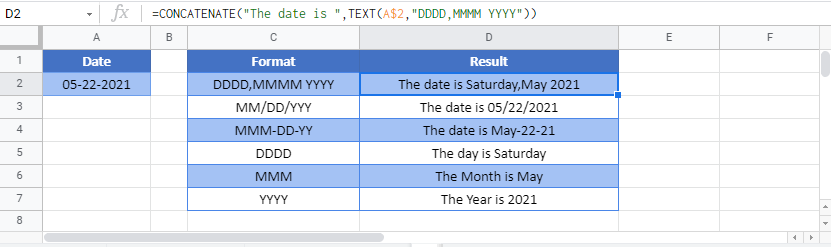### Excel Practice Worksheet

Practice Excel functions and formulas with our 100% free practice worksheets!